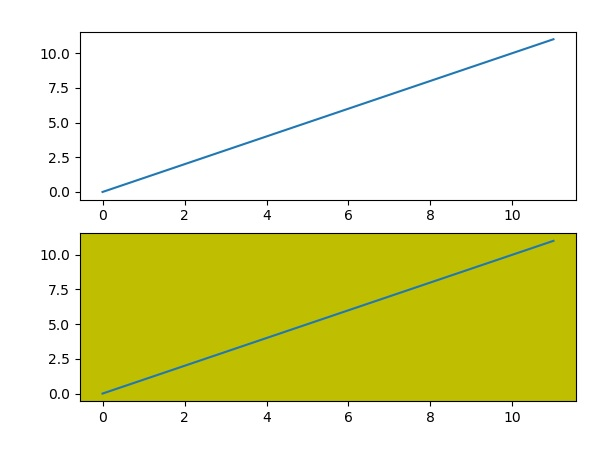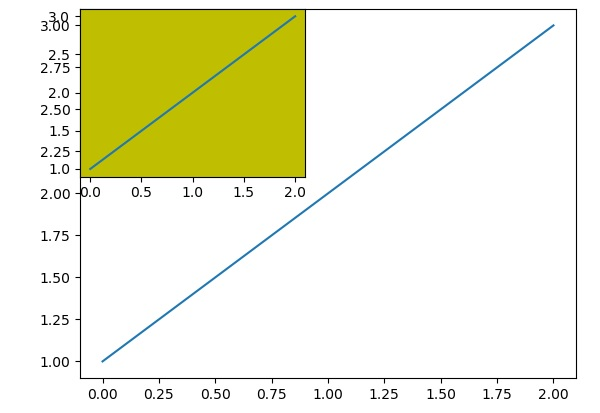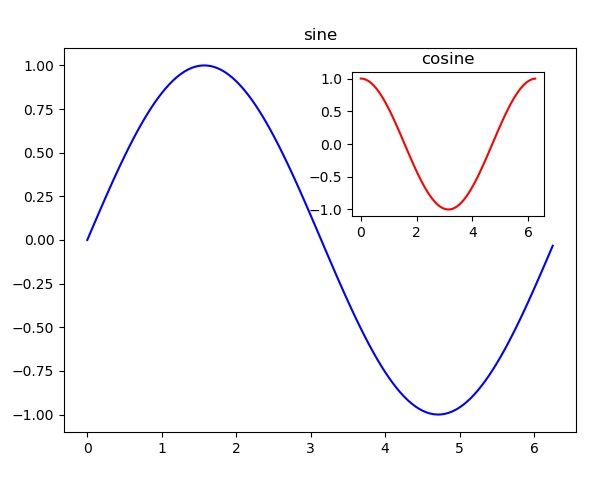# Matplotlib - Multiplots

In this chapter, we will learn how to create multiple subplots on same canvas.

The subplot() function returns the axes object at a given grid position. The Call signature of this function is −

```plt.subplot(subplot(nrows, ncols, index)
```

In the current figure, the function creates and returns an Axes object, at position index of a grid of nrows by ncolsaxes. Indexes go from 1 to nrows * ncols, incrementing in row-major order.Ifnrows, ncols and index are all less than 10. The indexes can also be given as single, concatenated, threedigitnumber.

For example, subplot(2, 3, 3) and subplot(233) both create an Axes at the top right corner of the current figure, occupying half of the figure height and a third of the figure width.

Creating a subplot will delete any pre-existing subplot that overlaps with it beyond sharing a boundary.

```import matplotlib.pyplot as plt
# plot a line, implicitly creating a subplot(111)
plt.plot([1,2,3])
# now create a subplot which represents the top plot of a grid with 2 rows and 1 column.
#Since this subplot will overlap the first, the plot (and its axes) previously
created, will be removed
plt.subplot(211)
plt.plot(range(12))
plt.subplot(212, facecolor='y') # creates 2nd subplot with yellow background
plt.plot(range(12))
```

The above line of code generates the following output −The add_subplot() function of the figure class will not overwrite the existing plot −

```import matplotlib.pyplot as plt
fig = plt.figure()
ax1.plot([1,2,3])
ax2.plot([1,2,3])
```

When the above line of code is executed, it generates the following output −You can add an insert plot in the same figure by adding another axes object in the same figure canvas.

```import matplotlib.pyplot as plt
import numpy as np
import math
x = np.arange(0, math.pi*2, 0.05)
fig=plt.figure()
axes1 = fig.add_axes([0.1, 0.1, 0.8, 0.8]) # main axes
axes2 = fig.add_axes([0.55, 0.55, 0.3, 0.3]) # inset axes
y = np.sin(x)
axes1.plot(x, y, 'b')
axes2.plot(x,np.cos(x),'r')
axes1.set_title('sine')
axes2.set_title("cosine")
plt.show()
```

Upon execution of the above line of code, the following output is generated −